U.S. Department of Transportation
1200 New Jersey Avenue, SE
Washington, DC 20590
202-366-4000

Federal Highway Administration Research and Technology
Coordinating, Developing, and Delivering Highway Transportation Innovations

 REPORT This report is an archived publication and may contain dated technical, contact, and link information
 Publication Number:  FHWA-HRT-12-030    Date:  August 2012
 Publication Number: FHWA-HRT-12-030 Date: August 2012

# Estimation of Key PCC, Base, Subbase, and Pavement Engineering Properties From Routine Tests and Physical Characteristics

PDF files can be viewed with the Acrobat® Reader®

# CHAPTER 5. MODEL DEVELOPMENT (11)

### Flexural Strength Model 1: Flexural Strength Based on Compressive Strength

The flexural strength model 1 provides the best correlation between compressive strength and flexural strength with the LTPP data. The model form utilizes the power equation. This model will be most useful for cases when the compressive strength of the PCC has been determined through a routine cylinder break.

This model can be expressed as follows: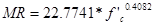#### Figure 172. Equation. Prediction model 6 for MR.

Where:

MR = Flexural strength, psi.

f'c,= Compressive strength determined at the same age, psi.

The regression statistics for this model are presented in table 33. The model was developed using 185 data points, and the prediction has an R2 value of 45.2 percent and an RMSE value of 69 psi. Table 34 provides details of the range of data used to develop the model. The confidence limits are both within acceptable ranges for both the regressed coefficients (i.e., limits are positive numbers). Figure 173 and figure 174 show the predicted versus measured plot and the residual plot, respectively.

#### Table 33. Regression statistics for flexural strength model based on compressive strength.

 Parameter Estimate Standard Error Approximate 95 Percent Confidence Limits a 22.7741 6.6362 9.6807 to 35.8674 b 0.4082 0.0338 0.3416 to 0.4748

The model statistics for table 33 are as follows:

• RMSE = 69 psi.
• R2 = 0.452 percent.
• N = 185.

#### Table 34. Range of data used for flexural strength model based on compressive strength.

 Parameter Minimum Maximum Average Compressive strength 1,770 10,032 5,431 Flexural strength 467 1,075 754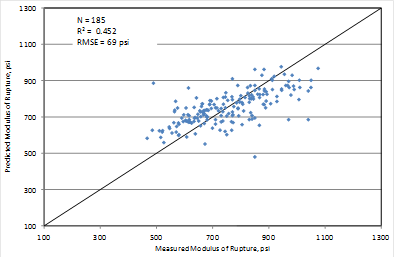#### Figure 173. Graph. Predicted versus measured values for flexural strength model based on compressive strength.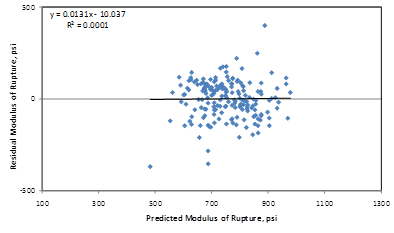#### Figure 174. Graph. Residuals errors for flexural strength model based on compressive strength.

Figure 175 shows a comparison of the power models used to validate the data and also to develop a new correlation. Note that the three power models (the new equation developed for this study as well as the validation models) provide close estimates (within 50 psi) in the 4,500- to 5,500-psi compressive strength range.

The ACI and PCA models are plotted for comparison. Also plotted in figure 175 are the raw data that were used in the model. Clearly, the ACI equation is very conservative for this data. It also has been found to give a conservative estimate for several large datasets that have been used in flexural strength model prediction. Conversely, the PCA model fits the LTPP data more closely. The reasons for this lack of fit of the current data with the previous models may be too many to fully explain. The data used in models from prior studies often came from mixes batched under controlled laboratory experiments and were typical of paving and structural concrete. The mixes used in the current model developed from LTPP data relies on only mixes proportioned for typical paving operations. Furthermore, the LTPP data used are from many projects widely dispersed around the United States. This in itself makes the models more robust than any previous data used to make similar correlations.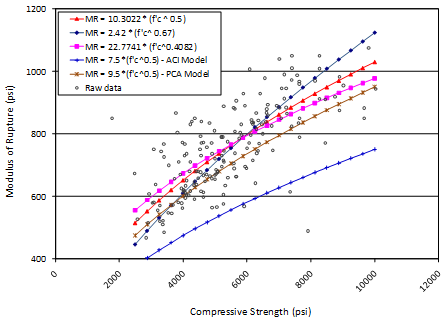#### Figure 175. Graph. Comparison of flexural strength models based on compressive strength.

The spread in the raw data about the prediction model in figure 175 indicates that there are factors other than compressive strength that influence the flexural strength of PCC. Among the various factors influencing flexural strength are the mix design parameters and age of the concrete. These variables were considered in the other models developed in this study.

### Flexural Strength Model 2: Flexural Strength Based on Age, Unit Weight, and w/c Ratio

Flexural strength model 2 provides a correlation between flexural strength and mix design parameters, specifically the unit weight and w/c ratio. Age is also a parameter in this model, which helps reduce some of the variability seen in the prediction relative to the predictions shown in figure 175. This model will be most useful for cases when the compressive strength of the PCC is not determined but mix design information is available. Also, the user has the option of predicting the 28-day strength value for design or estimating the strength at traffic opening time.

This model can be expressed as follows: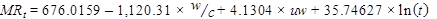#### Figure 176. Equation. Prediction model 7 for MRt.

Where:

MRt = Flexural strength at age t years, psi.

w/c = Water to cement ratio.

uw = Unit weight, lb/ft3.

t = Pavement age, years.

The regression statistics for this model are presented in table 35. The model was developed using 62 data points, and the prediction has an R2 value of 61.1 percent and an RMSE value of 69 psi. Table 36 provides details of the range of data used to develop the model. Figure 177 and figure 178 show the predicted versus measured plot and the residual plot, respectively.

#### Table 35. Regression statistics for flexural strength model based on age, unit weight, and w/c ratio.

 Variable DF Estimate Standard Error t-value Pr > t VIF Intercept 1 676.0159 277.7887 2.43 0.0181 0 w/c 1 -1,120.31 141.3573 -7.93 < 0.0001 1.00591 Unit weight 1 4.1304 1.88934 2.19 0.0329 1.00311 Ln(age) 1 35.74627 8.78516 4.07 0.0001 1.00619

The model statistics for table 35 are as follows:

• RMSE = 91 psi.
• R2 = 0.6111 percent.
• N = 62.

#### Table 36. Range of data used for flexural strength model based on age, unit weight, and w/c ratio.

 Parameter Minimum Maximum Average w/c ratio 0.27 0.58 0.40 Unit weight 124 151 142 Pavement age 0.0384 1.0000 0.3169 Flexural strength 467 978 742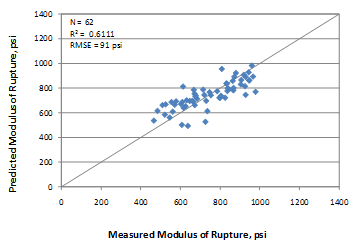#### Figure 177. Graph. Predicted versus measured values for flexural strength model based on age, unit weight, and w/c ratio.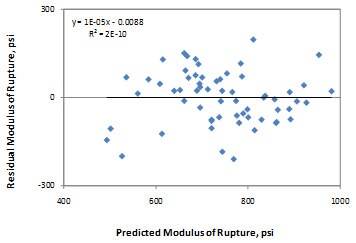### Flexural Strength Model 3: Flexural Strength Based on Age, Unit Weight, and CMC

The data used in the previous model also provided a good correlation by replacing the w/c ratio parameter with CMC. The model is expressed as follows: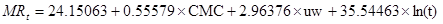#### Figure 179. Equation. Prediction model 8 for MRt.

Where:

MRt = Flexural strength at age t years, psi.

CMC = Cementitious materials content, lb/yd3.

uw = Unit weight, lb/ft3.

t = Pavement age, years.

The regression statistics for this model are presented in table 37. The model was developed using 62 data points, and the prediction has an R2 value of 70.2 percent and an RMSE value of 80 psi. Table 38 provides details of the range of data used to develop the model. Figure 180 and figure 181 show the predicted versus measured plot and the residual plot, respectively.

Figure 182 to figure 185 present the sensitivity of the mix design-based flexural strength models to CMC, w/c ratio, unit weight, and age. Figure 182 and figure 183 show that prediction models in figure 176 and figure 179 do not show any sensitivity to CMC and w/c ratio. For typical values of these parameters, the flexural strength prediction from these two models could show a difference of about 200 psi for extreme values of w/c ratios. However, within a typical range of 0.35 to 0.45, the flexural strength prediction is within 50 psi. Similar trends are evident for the w/c ratio parameter. Therefore, if all details about a mix design are available, it is highly recommended that both models be used to predict flexural strength so that the user has a fair estimate of the MR range. Figure 184 shows that the predictions are close from both models. Likewise, figure 185, which is more or less a flexural strength gain model for a typical mix design, shows close predictions from both models.

#### Table 37. Regression statistics for flexural strength model based on age, unit weight, and CMC.

 Variable DF Estimate Standard Error t-value Pr > t VIF Intercept 1 24.15063 236.7606 0.1 0.9191 0 CMC 1 0.55579 0.05563 9.99 < 0.0001 1.01522 Unit weight 1 2.96376 1.66087 1.78 0.0796 1.01253 Ln(age) 1 35.54463 7.68504 4.63 < 0.0001 1.00573

The model statistics for table 37 are as follows:

• RMSE = 80 psi.
• R2 = 0.7023 percent.
• N = 62.

#### Table 38. Range of data used for flexural strength model based on age, unit weight, and CMC.

 Parameter Minimum Maximum Average CMC 388 936 668 Unit weight 124 151 142 Pavement age 0.0384 1.0000 0.3169 Flexural strength 467 978 742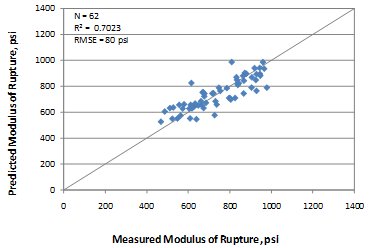#### Figure 180. Graph. Predicted versus measured values for flexural strength model based on age, unit weight, and CMC.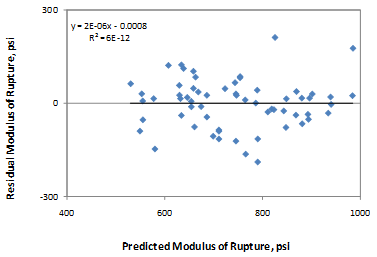#### Figure 181. Graph. Residuals errors for flexural strength model based on age, unit weight, and CMC.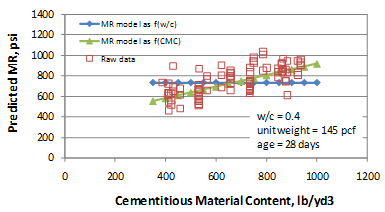#### Figure 182. Graph. Sensitivity of flexural strength predictions to CMC.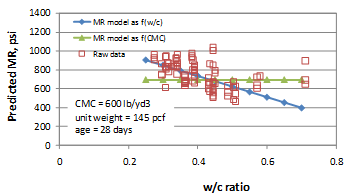#### Figure 183. Graph. Sensitivity of flexural strength predictions to w/c ratio.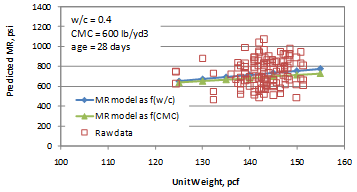#### Figure 184. Graph. Sensitivity of flexural strength predictions to unit weight.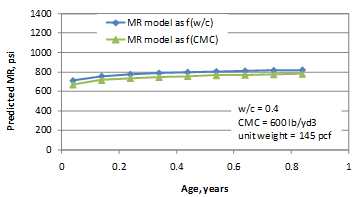#### Figure 185. Graph. Sensitivity of flexural strength predictions to age.

Federal Highway Administration | 1200 New Jersey Avenue, SE | Washington, DC 20590 | 202-366-4000
Turner-Fairbank Highway Research Center | 6300 Georgetown Pike | McLean, VA | 22101